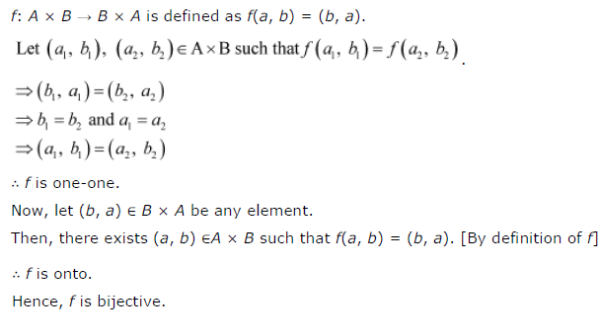# Let A and B be sets. Show that f : A x B → B x A such that f(a,b) = (b,a) is bijective function

Let A and B be sets. Show that f : A x B → B x A such that f(a,b) = (b,a) is bijective function.